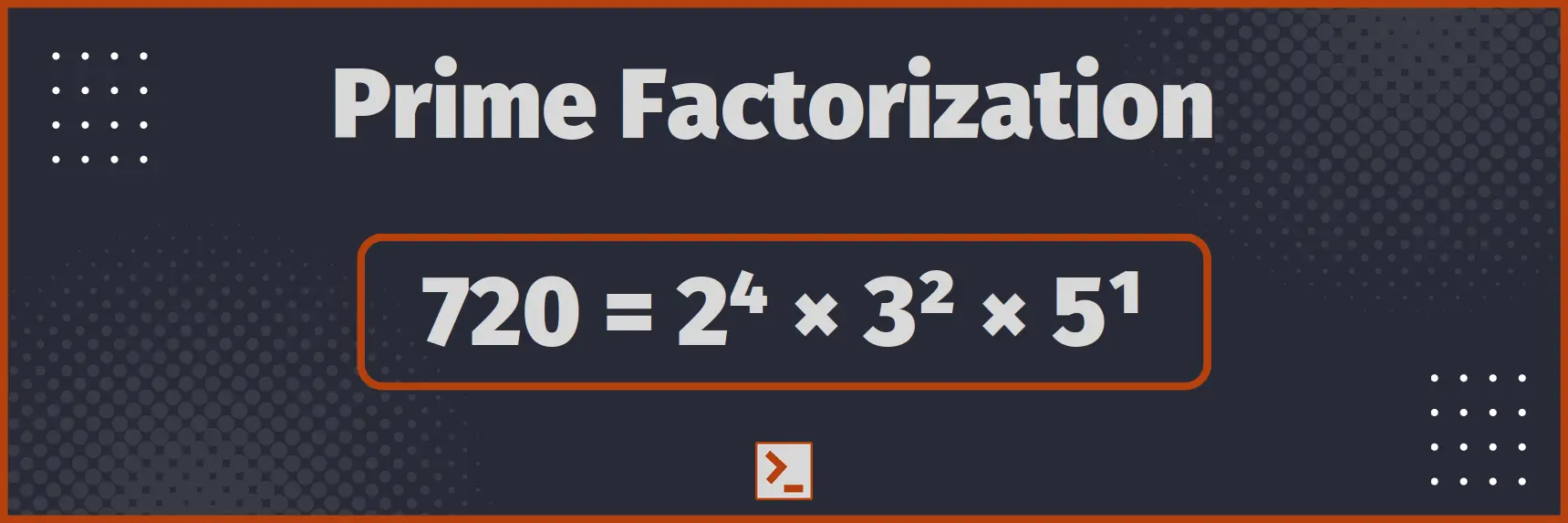# Prime Factorization of a natural number

And a program in Rust to list all the prime factors of a natural number

## What is Prime Factorization of a number

Prime Factorization of a natural number is splitting the number into its factors, which are prime numbers. It can also be understood as writing a natural number as the product of prime numbers.

For Example : 100 = 22 × 52## Approach

Using BTreeMap

1. While number is divisible by 2, add 2 to prime factors and divide the number by 2.
2. Now, number is odd number. So, start from 3, and go till square root of number, perform the step 3.
3. While i divides number, simply add i to prime factors.
4. If the number, after loop, does not become 1, number that is left, itself is a prime number.

## Program

Program using above approach is given below :

```pub fn prime_factorization(mut number:i128) -> BTreeMap<i128, i128> {    let mut prime_factors: BTreeMap<i128, i128> = BTreeMap::new();
// Step 1 : Divide by 2    let mut freq:i128 = 0;
// You can use number % 2 == 0 also,    // but this method is much more efficient    while number&1 == 0 {        number >>=1;        // Again, You can use number /= 2 also,        // but this is much more efficient        freq+=1;    }
if freq > 0 {        prime_factors.insert(2, freq);    }
// Step 2 : start from 3, and go till square root of number    let mut i = 3;    while i*i <= number {
// Step 3 : Check if i is factor of number        if number%i==0 {            freq = 0;            while number%i==0 {                number/=i;                freq+=1;            }            prime_factors.insert(i, freq);        }        i+=2;    }
// Step 4 : Check if number become 1 or not    if number > 1 {        prime_factors.insert(number, 1);    }
return prime_factors;}```

Program With Driver Code

```use std::collections::BTreeMap;use std::io::stdin;
fn take_int() -> i128 {    let mut input = String::new();    stdin().read_line(&mut input).unwrap();    input.trim().parse().unwrap()}
// Magic starts here
pub fn prime_factorization(mut number:i128) -> BTreeMap<i128, i128> {    let mut prime_factors: BTreeMap<i128, i128> = BTreeMap::new();
// Step 1 : Divide by 2    let mut freq:i128 = 0;
// You can use number % 2 == 0 also,    // but this method is much more efficient    while number&1 == 0 {        number >>=1;        // Again, You can use number /= 2 also,        // but this is much more efficient        freq+=1;    }
if freq > 0 {        prime_factors.insert(2, freq);    }
// Step 2 : start from 3, and go till square root of number    let mut i = 3;    while i*i <= number {
// Step 3 : Check if i is factor of number        if number%i==0 {            freq = 0;            while number%i==0 {                number/=i;                freq+=1;            }            prime_factors.insert(i, freq);        }        i+=2;    }
// Step 4 : Check if number become 1 or not    if number > 1 {        prime_factors.insert(number, 1);    }
return prime_factors;}
// Driver Code Starts
pub fn main() {    // Take input    let number = take_int();
// Call Our function    let prime_factors = prime_factorization(number);
// Print result    for (key, value) in prime_factors {        println!("{} appears {} time", key, value);    }}```

Input

720

Output

2 appears 4 time
3 appears 2 time
5 appears 1 time

Time Complexity : O( sqrt(n) )
Space Complexity : O( log(n) )

Note : We can print the number instead of storing into the BtreeMap, to reduce Space Complexity to O(1) but it is rarely useful. BTreeMap or vector of prime factors is far more useful in real applications, so I will demonstrate BTreeMap, instead of printing directly.

You can also modify the code to use HashMap or Vector or BTreeSet or HashSet, according to your requirement.

## Conclusion

Prime Factorization of a natural number is splitting the number into the product of prime numbers. It is used for various applications.

In this article, we made a function to generate all the prime factors of a number and store them with their respective exponents. Here is the function for easy access

```pub fn prime_factorization(mut number:i128) -> BTreeMap<i128, i128> {    let mut prime_factors: BTreeMap<i128, i128> = BTreeMap::new();    let mut freq:i128 = 0;    while number&1 == 0 {        number>>=1;        freq+=1;    }    if freq > 0 { prime_factors.insert(2, freq);}    let mut i = 3;    while i*i <= number {        if number%i==0 {            freq = 0;            while number%i==0 {                number/=i;                freq+=1;            }            prime_factors.insert(i, freq);        }        i+=2;    }    if number > 1 {prime_factors.insert(number, 1);}
return prime_factors;}```

Above program runs on O( sqrt (n) ) time complexity. We can also optimize the code using sieve method, which we will discuss later.

Thank You